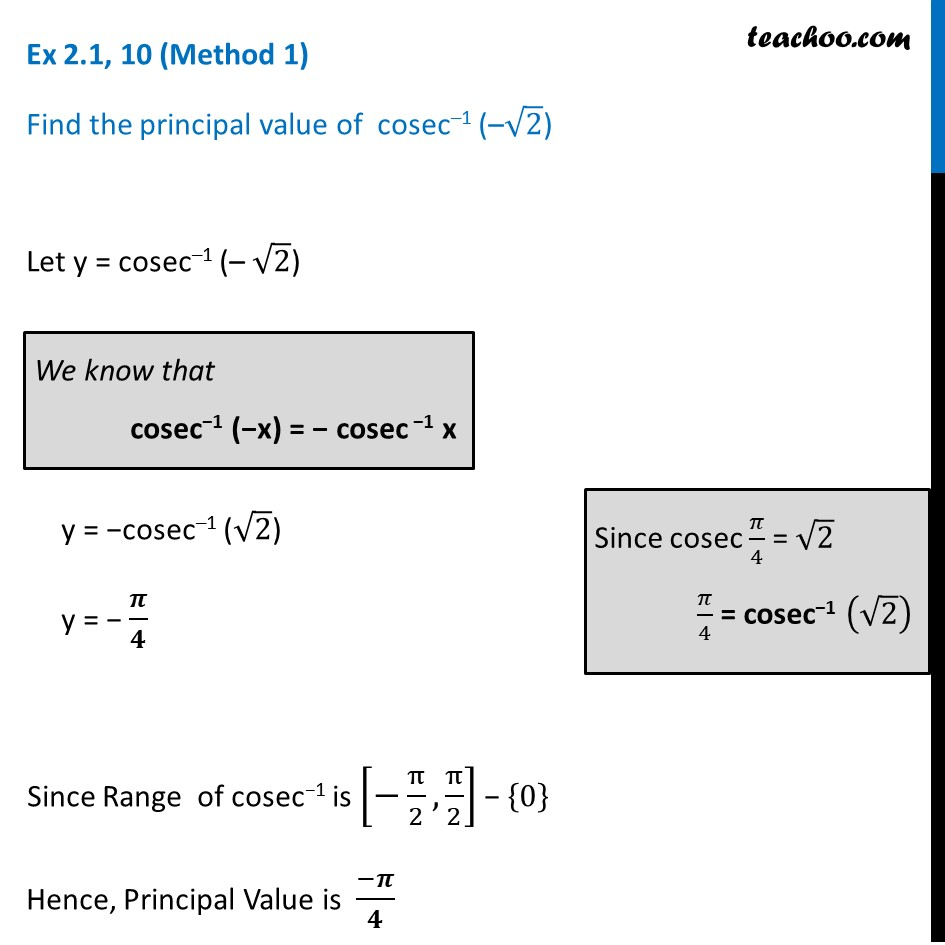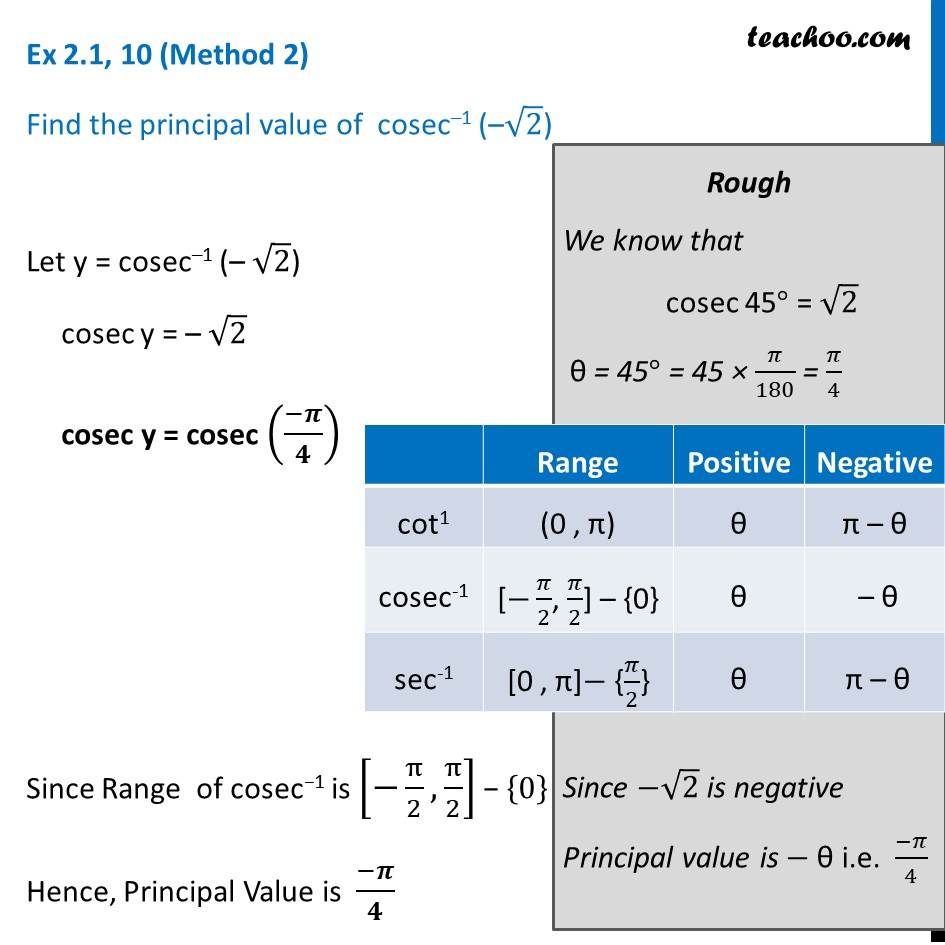Finding principal value

Chapter 2 Class 12 Inverse Trigonometric Functions
Concept wiseLearn in your speed, with individual attention - Teachoo Maths 1-on-1 Class

### Transcript

Ex 2.1, 10 (Method 1) Find the principal value of cosec–1 (–√2) Let y = cosec–1 (– √2) y = −cosec–1 (√2) y = − 𝝅/𝟒 Since Range of cosec−1 is [−π/2,π/2] − {0} Hence, Principal Value is (−𝝅)/𝟒 We know that cosec−1 (−x) = − cosec −1 x Since cosec 𝜋/4 = √2 𝜋/4 = cosec−1 (√2) Ex 2.1, 10 (Method 2) Find the principal value of cosec–1 (–√2) Let y = cosec–1 (– √2) cosec y = – √2 cosec y = cosec ((−𝝅)/𝟒) Since Range of cosec−1 is [−π/2,π/2] − {0} Hence, Principal Value is (−𝝅)/𝟒 Rough We know that cosec 45° = √2 θ = 45° = 45 × 𝜋/180 = 𝜋/4 Since −√2 is negative Principal value is − θ i.e. (−𝜋)/4